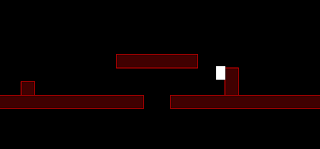# Nerdy Gentleman Games

## Platform Progress - Collision Detection Sucks

9/28/2011 03:27:00 pm Posted by LachlanThose red boxes? The things you can collide with. The white box?  You. Simple looking I know - but slowly getting there
I've been wrestling with collision detection quite extensively over the last week and a bit (and a bit more). I've had varying things working, but they either partially work by colliding well and not necessarily putting the actor back quite where it needs to go, or getting stuck in walls, and generally not being able to register as a collision when they're just touching - or freezing the box if it could.

I've ended up using a simplified case of the technique in http://www.metanetsoftware.com/technique/tutorialA.html - it's the one they use for 'N'. If I end up adding ramps or anything I may have to do it in more detail, but it seems to work. However, the code wasn't liberal enough in detection of collision where the platform and actor are just touching - without overlap. So, I've added a simple, more liberal check at the end. Code is below, if it's your sort of thing or you need help doing something similar.

1 void Feature::TotalCollision(Actor* actor, float dt)
2     //Method described in this... partially
3     //http://www.metanetsoftware.com/technique/tutorialA.html#section5
4 {
5     //These shouldn't be using typecast x1() but instead x - w/2 or something - Maybe
6     //This may change if features start being defined by x, w, y & h rather then
7     //w and h being calculated and the feature being defined by (x1, y1) and (x2, y2).
8     float left_a = actor->x() - (actor->w() / 2);
9     float left_f = (float)x1();
10     float right_a = actor->x() + (actor->w() / 2);
11     float right_f = (float)x2();
12     float top_a = actor->y() - (actor->h() / 2);
13     float top_f = (float)y1();
14     float bottom_a = actor->y() + (actor->h() / 2);
15     float bottom_f = (float)y2();
16     float midx_f = (left_f + right_f) / 2;
17     float midy_f = (top_f + bottom_f) / 2;
18
19     //bool colliding = Collides(left_a, right_a, top_a, bottom_a, left_f, right_f, top_f, bottom_f);
20
21     bool colliding = true;
22
23     if (bottom_a <= top_f) { colliding = false; };
24     if (top_a >= bottom_f) { colliding = false; };
25     if (right_a <= left_f) { colliding = false; };
26     if (left_a >= right_f) { colliding = false; };
27
28
29     if (colliding)
30     {
31         float x_overlap = (actor->x() > midx_f) ? left_a - right_f : right_a - left_f;
32         float y_overlap = (actor->y() > midy_f) ? top_a - bottom_f : bottom_a - top_f;
33         if (std::abs(x_overlap) > std::abs(y_overlap))
34         {
35             actor->set_y(actor->y() - y_overlap);
36             actor->set_dy(0);
37             actor->set_v_collided (true);
38         }
39         else
40         {
41             actor->set_x(actor->x() - x_overlap);
42             actor->set_dx(0);
43             actor->set_h_collided (true);
44         }
45     }
46
47     //These fill the special case uncovered by the above where there needs to be a collision
48     //for whether or not we can jump or not, but the code doesn't need to move the actor
49     if
50     (
51         ((bottom_a == top_f) || (bottom_f == top_a)) &&
52         !((left_a > right_f) || (right_a < left_f))
53     )
54     {
55         actor->set_v_collided(true) ;
56     } ;
57
58     if
59     (
60         ((right_a == left_f) || (right_f == left_a)) &&
61         !((top_a > bottom_f) || (bottom_a < top_f))
62     )
63     {
64         actor->set_h_collided(true) ;
65     } ;
66
67 }

By the way - if any of you know a good, easy way to highlight code for blogs - preferably an online utility, I'd really appreciate knowing it.

Lachlan